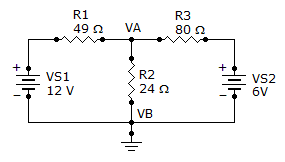# Electrical Engineering - Branch, Loop and Node Analyses - Discussion

Discussion Forum : Branch, Loop and Node Analyses - General Questions (Q.No. 1)
1.
Find the node voltage VA.6 V
12 V
4.25 V
3 V
Explanation:
No answer description is available. Let's discuss.
Discussion:
39 comments Page 1 of 4.

Pewds said:   11 months ago
@All.

Here is the soltion.
Millman's Theorem.
(Î£ E/R)/Î£ 1/R.

Vab = (12/49 + 0/24 +6/80) / (1/49 + 1/24 + 1/80).
Vab = 4.2896 V.
(5)

Sharathkumar said:   2 years ago
((Va-12)/49) + (Va/24) + (Va-6)/80))=0
((24Va-288 + 49Va)/1176) + (Va-6)/80))=0
1920Va-2340 + 3920Va + 1176Va-7056=0
7016Va-30096 =0,
7016Va = 30096,
Va = 30096/7016,
= 4.28.
(5)

Nami-Swan Jyv said:   2 years ago
KCL: Iin = Iout.
I1 + I3 = I2.
I1 - I2 + I3 = 0.

Nodal Analysis:

(Start node minus End node)/Resistor.

((V1- Va)/R1) - ((Va-0)/R2) +((V2-Va)/R3) = 0.

Shift Solve;

Va= 4.28v.
or
Va = 4.25v.
(2)

Abyssinia said:   3 years ago
Thanks all for explaining.
(1)

Adel saeed said:   3 years ago
Thanks @Abdullah.

Balu said:   4 years ago
Thanks all for giving the solution.

Rakesh. Parida said:   4 years ago
Thanks all for explaining it.

Yamanappa said:   4 years ago
((Vs1 - Va) /R1)+((Vs2 - Va)/R3)) = Va/R2.
(1)

Dinesh sah said:   5 years ago